Click to Chat

1800-1023-196

+91-120-4616500

CART 0

• 0

MY CART (5)

Use Coupon: CART20 and get 20% off on all online Study Material

ITEM
DETAILS
MRP
DISCOUNT
FINAL PRICE
Total Price: Rs.

There are no items in this cart.
Continue Shopping• Complete Physics Course - Class 11
• OFFERED PRICE: Rs. 2,968
• View Details

```Motion in a Vertical Circle

Table of Content

Critical Velocity

Related Resources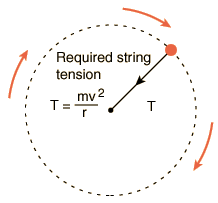Whenever a body is released from height, it travels vertically downward towards the surface of earth. This is due to the force of gravitational attraction exterted on body by the earth. The acceleration produced by this force is called acceleration due to gravity and is denoted by ‘g’. Value of ‘g’ on the surface of earth is taken to the 9.8 m/s2 and it is same for all the bodies. It means all bodies (whether an iron ball or a piece of paper), when dropped (u=0) from same height should fall with same rapidity and should take same time to reach the earth. Our daily observation is contrary to this concept. We find that iron ball falls more rapidlly than piece of paper. This is due to the presence of air which offers different resistance to them. In the absence of air both would have taken same time to reach the surface of earth.

A particle of mass m is attached to a light and inextensible string. The other end of the string is fixed at O and the particle moves in a vertical circle of radius r equal to the length of the string as shown in the figure.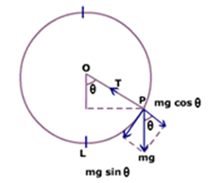Consider the particle when it is at the point P and the string makes an angle θ with vertical. Forces acting on the particle are:

T = tension in the string along its length, and

mg = weight of the particle vertically downward.

Hence, net radial force on the particle is FR = T - mg cos θ

=> T - mg cos θ = mv2/R

=> T = mv2/R + mg cos θ

Since speed of the particle decreases with height, hence tension is maximum at the bottom, where cos θ = 1 (as θ = 0).

=> Tmax = mv2/R + mg; Tmin = mv'2/R - mg (at the top)

Here,  v' = speed of the particle at the top.

Critical VelocityIt is the minimum velocity given to the particle at the lowest point to complete the circle.

The tendency of the string to become slack is maximum when the particle is at the topmost point of the circle.

At the top, tension is given by T = mvT2/R - mg, where vT = speed of the particle at the top.

=>   mvT2/R  = T + mg

For vT to be minimum, T ≈ 0 => vT = √gR

If vB be the critical velocity of the particle at the bottom, then from conservation of energy

Mg(2R) +  1/2 mvT2  = 0 + 1/2 mvB2

As vT = √gR => 2mgR + 1/2 mgR = 1/2 mvB2

Thus,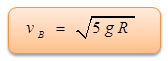Note: In case the particle is attached with a light rod of length l, at the highest point its minimum velocity may be zero. Then, the critical velocity is 2√gl.

Problem 1:-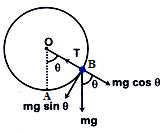A heavy particle hanging from a fixed point by a light inextensible string of length l is projected horizontally with speed √(gl). Find the speed of the particle and the inclination of the string to the vertical at the instant of the motion when the tension in the string is equal to the weight of the particle.

Solution :-

Let tension in the string becomes equal to the weight of the particle when particle reaches the point B and deflection of the string from vertical is θ. Resolving mg along the string and perpendicular to the string, we get net radial force on the particle at B, i.e.

FR = T - mg cos θ                                ... (i)

If vB be the speed of the particle at B, then

FR = mvB2/l                                     ... (ii)

From (i) and (ii), we get,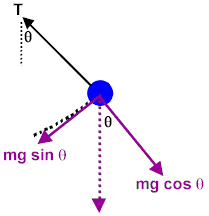T - mg cos θ = mvB2/l                       ... (iii)

Since at B, T = mg => mg(1 - cos θ) = mvB2/l

=> vB2 = gl (1 - cos θ)                       ... (iv)

Conserving the energy of the particle at points A and B, we have

1/2mvA2 = mgl (1 - cos θ) + 1/2mvB2

where vA=√gl and vB=√gl(1-cos θ) => gl =2gl(1-cos θ) + gl(1-cos θ)

=> cos θ = 2/3                                 ... (v)

Putting the value of cos q in equation (iv), we get v = √gl/3

A particle move along a circle of radius (20/π) m with constant tangential acceleration. If the speed of the particle is 80 m/s at the end of the second revolution after motion has begun, then find out the tangential acceleration.

Solution :-

Let tangential acceleration, at = a

Then,

v =  √2ad           (d = distance travelled)

So, a = v2/ad = v2/2(2πR) = v2/(4πR)

Thus, a = (80)2/[(4π) (20/π)] = 80 m/s2

From the above observation we conclude that, the tangential acceleration would be 80 m/s2.As the object goes round the circle the tension in the string varies being greatest at the bottom of the circle and least at the top.

As the object goes round the circle the tension in the string varies being least at the top of the circle.

If the string is to break it will be at the bottom of the path where it has to not only support the object but also pull it up out of it straight-line path.

The condition for the object to execute a complete vertical circle without the string is independent of the mass of the object.

It is slightly easier to cause an object attached to the end of a rigid rod to execute a vertical circle than it is to cause an object attached to the end of a string to execute the same circle.Question 1:-

A stone released with zero velocity from the top of a tower reaches the ground in 4 seconds, the height of the tower is about

(a) 20 m              (b) 40 m

(c) 80 m              (d) 16 m

Question 2:-

An iron ball and a wooden ball of same radius are released from a height h in vacuum. The time taken by both of them to reach the ground are

(a) roughly equal         (b) exactly equal

(c) unequal                 (d) equal only at equator

Question 3:-

A body is thrown upwards from ground covers equal distances in 4th and 7th second. With what initial velocity the body was projected?

(a) 20 m/s           (b) 50 m/s

(c) 30 m/s           (d) 10 m/s

Question 4:-

Two balls are dropped from same height at 1 second interval of time. The separation between the two balls after 3 seconds of the drop of the first ball is:

(a) 50 m            (b) 40 m

(c) 35 m            (d) 25 mQ.1
Q.2
Q.3
Q.4

c

b

b

d

Related Resources

You might like to refer Kinematics.

For getting an idea of the type of questions asked, refer the  Previous Year Question Papers.

```### Course Features

• 101 Video Lectures
• Revision Notes
• Previous Year Papers
• Mind Map
• Study Planner
• NCERT Solutions
• Discussion Forum
• Test paper with Video Solution